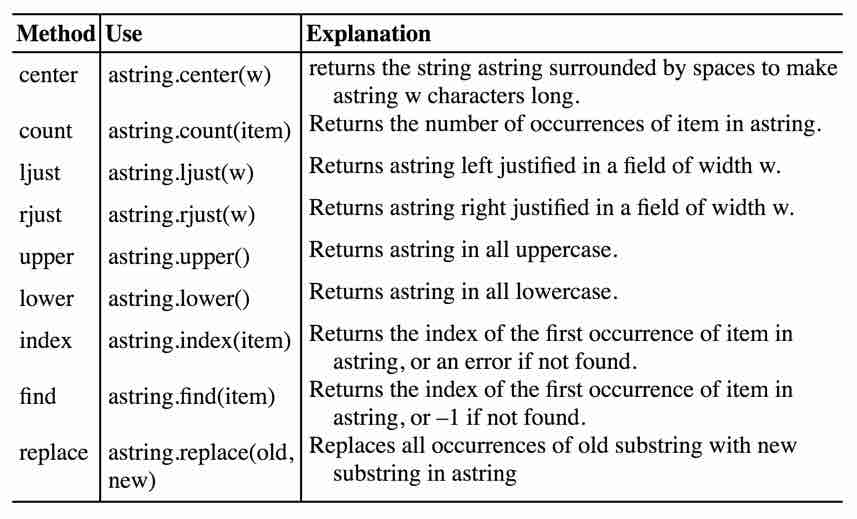# Lecture 7

2/6/2018

## String Methods

Table 3.2 in the text gives a list of methods you can use to obtain information about a string or to create a new string as a modification of an existing string. These methods all leave the original string unaltered. Here is a copy of the table.There is a more complete list of string methods at https://docs.python.org/3/library/stdtypes.html#string-methods

## Exercise

Create a string containing the name Julius Caesar. Use this string for all of the following exercises.

Use an appropriate method to center this string in a field of 30 characters.

s = 'Julius Caesar'
s2 = s.center(30)
print(s)
print(s2)
## Julius Caesar
##         Julius Caesar

## Exercise

Print the name right-justified in a field of 20 characters.

s = 'Julius Caesar'
s2 = s.rjust(20)
print(s)
print(s2)
## Julius Caesar
##        Julius Caesar

## Exercise

Print the name centered in a field of 5 characters. What happens when the field is too small?

s = 'Julius Caesar'
s2 = s.center(5)
print(s)
print(s2)
## Julius Caesar
## Julius Caesar

## Exercise

Turn the name into an expletive by inserting ‘Bleeping’ as a middle name.

s = 'Julius Caesar'
s2 = s.replace(" "," Bleeping ")
print(s)
print(s2)
## Julius Caesar
## Julius Bleeping Caesar

## Exercise

Use the safe method to find the index of the character ‘v’ in the name.

s = 'Julius Caesar'
idx = s.find("v")
print(s)
print(idx)
## Julius Caesar
## -1

## Exercise

Write a program that could be used to reverse the order in which a name is written. It should begin with a name written like ‘Julius Caesar’. It should Rewrite the name in a form like ‘Caesar, Julius’. The program should work with any combination of first and last names. Use ‘Julius Caesar’ as a test case.

Begin by finding the index of the blank space separating the two names. What do you do next? Use slicing and concatenation.

s = 'Julius Caesar'
idx = s.find(" ")
fname = s[:idx]
lname = s[idx+1:]
name = lname + ", " + fname
print(name)
## Caesar, Julius

## ASCII

Review the material at https://en.wikipedia.org/wiki/ASCII

## Writing Numbers

Ordinarily we write numbers in the decimal system. Think about them as coefficients of a polynomial in powers of 10.

Take the simple number $$123$$.

we could write this as $1 * 10^2 + 2 * 10^1 + 3 * 10^0$

## Exercise

Write the number 5,426 in this polynomial format.

$5*10^3+4*10^2+2*10^1+6*10^0$

## Thoughts on Numbers

With decimal numbers, the valid symbols are 0 through 9. There is no symbol for 10 itself.

We could choose some other number, such as 2 to play the role that 10 plays with decimal numbers. Binary numbers use 2 for this purpose, so the number 2 has no symbol in the binary system. The valid symbols are 0 and 1.

## Exercise

How do you interpret the binary number 10?

$1*2^1+ 0*2^0$

## Exercise

Write a python program to show the values of all powers of 2 from 0 to 10 inclusive.

for i in range(11):
print(i,2**i)
## 0 1
## 1 2
## 2 4
## 3 8
## 4 16
## 5 32
## 6 64
## 7 128
## 8 256
## 9 512
## 10 1024

## Exercise

Interpret the binary number 110111?

$1*2^5+1*2^4+0*2^3+1*2^2+1*2^1+1*2^0$ This can be re-written using our knowledge of powers of 2 as

$1*32+1*16+0*8+1*4+1*2+1*1=55$

## Exercise

Write a python function that accepts binary numbers as character strings and returns a decimal value.

def bindec(bs):
l = len(bs)
ans = 0
power = l - 1
for i in range(l):
ans = ans + int(bs[i]) * 2**power
power = power - 1
return ans
print(bindec("110111"))

## 55

## Convert Decimal to Binary

Take 125 as an example. What is the largest power of 2 that does not exceed 125?

Note that $$2^6 = 64$$ and $$2^7=128$$. Therefore the leading term in our polynomial will be $$1*2^6$$.

The remaining sum of 61 must come from lower order terms. What is the largest power of 2 that does not exceed 61? Clearly $2^5 = 32 We need to add the term $$1*2^5$$ to our polynomial. The sum of the terms we’ve accepted so far is $$96$$, which leaves us looking for another $$29$$. The next term we accept is $$2^4 = 16$$. That leaves us looking for $$13$$. We accept $$2^3=8$$, which leaves us looking for $$5$$. We accept $$2^2=4$$, which leaves us looking for $$1$$. We can’t accept $$2^1=2$$. We do accept $$2^0=1$$. We now have all of the coefficients we need and we can write the binary representation. 1111101 Let’s test it. def bindec(bs): l = len(bs) ans = 0 power = l - 1 for i in range(l): ans = ans + int(bs[i]) * 2**power power = power - 1 return ans print(bindec("1111101"))  ## 125 ## Exercise How do you write 13 as a binary number? ## Answer 1101 ## Convert Decimal to Binary Take 125 as an example. What is the largest power of 2 that does not exceed 125? Note that $$2^6 = 64$$ and $$2^7=128$$. Therefore the leading term in our polynomial will be $$1*2^6$$. The remaining sum of 61 must come from lower order terms. What is the largest power of 2 that does not exceed 61? Clearly$2^5 = 32

We need to add the term $$1*2^5$$ to our polynomial. The sum of the terms we’ve accepted so far is $$96$$, which leaves us looking for another $$29$$.

The next term we accept is $$2^4 = 16$$. That leaves us looking for $$13$$.

We accept $$2^3=8$$, which leaves us looking for $$5$$.

We accept $$2^2=4$$, which leaves us looking for $$1$$.

We can’t accept $$2^1=2$$.

We do accept $$2^0=1$$. We now have all of the coefficients we need and we can write the binary representation. 1111101 Let’s test it.